×#### Thank you for registering.

One of our academic counsellors will contact you within 1 working day.

Click to Chat

1800-1023-196

+91-120-4616500

CART 0

• 0

MY CART (5)

Use Coupon: CART20 and get 20% off on all online Study Material

ITEM
DETAILS
MRP
DISCOUNT
FINAL PRICE
Total Price: Rs.

There are no items in this cart.
Continue Shopping• Complete Physics Course - Class 11
• OFFERED PRICE: Rs. 2,968
• View Details

```Magnetic Moment

Table of Content

Magnetic Dipoles

External Magnetic Field produced by a Magnetic Dipole Moment

Internal Magnetic Field of a Dipole

Calculation of Magnetic Moment of a Bar Magnet

Related Resources

Magnetic Moment is an important topic in the Physics syllabus of IIT JEE. The magnetic moment of a magnet is the quantity that finds the force that a magnet is capable of exerting on electric currents and the torque that the magnetic field ill exert on it. Magnetic moments are exhibited by numerous things including a loop of electric current, a bar magnet, an electron, a molecule or a planet.

As we have discussed in magnetic fields, magnetic moment is also a vector which as it has magnitude as well as a direction. The direction of the magnetic moment points from the south to North Pole of a magnet. The magnetic field produced by a magnet is proportional to its magnetic moment as well. The term magnetic moment refers to the system’s magnetic dipole moment. The dipole component of an object's magnetic field is symmetric about the direction of its magnetic dipole moment, and decreases as the inverse cube of the distance from the object.

Magnetic moment, which is also called a magnetic dipole moment, is a measure of the object's tendency to align with a magnetic field. Its positive direction depends on the way the object responds to the magnetic field. The objects have a tendency to place themselves in such a way that the magnetic moment vector becomes parallel to the magnetic field lines. Magnetic moment is basically generated in two ways including:

The Motion of Electric Charge

Spin Angular Momentum

For example, a loop of wire with a current running through it will have a magnetic moment proportional to the current and area of the loop pointing in the direction of your right thumb if your fingers are curling in the direction of the current.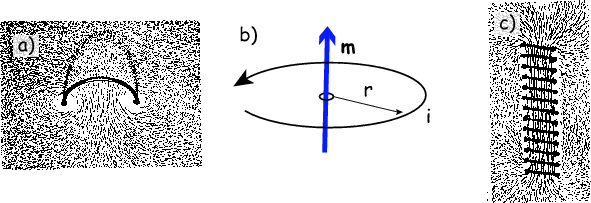The above figure shows how the magnetic field is generated by the current flowing in the loop. The (b) part of the figure shows a current loop with current i and area producing a magnetic moment m. the last figure depicts the magnetic fields of loops arranged as a solenoid which shows the total contribution of the individual loops.

Magnetic Dipoles

A magnetic dipole defines the limit of either a current loop or a pair of poles since the dimensions of the source are reduced to zero, whereas the moment is kept as constant. When these limits apply to fields far away from sources, they are the same. But, the two models are different for the internal field. Magnetic dipole moment physics, IIT JEE is an important topic for the JEE aspirants as it lays the groundwork for various other topics.

External Magnetic Field produced by a Magnetic Dipole MomentAny system that has a net magnetic dipole moment m produces a dipolar magnetic field. Although, the net magnetic field produced by the system may exhibit higher order multipole components, but they fall out very rapidly with distance and at last, only the dipolar component will dominate the magnetic field of the system at distances far away from it.

Internal Magnetic Field of a Dipole

Both the models for a dipole give the same result for the magnetic field far from the source. But the predictions inside the source region are different. The magnetic field between poles is in the opposite direction to the magnetic moment. it is apparent form the figure that the limits of these fields must also be different as the sourecs ultimately reduce to zero size.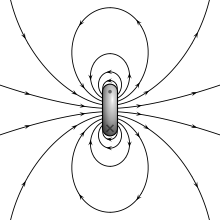Calculation of Magnetic Moment of a Bar Magnet

(a)   If a magnet of length l and magnetic moment M is bent in the form of a semicircular are then its new magnetic moment will be M' = 2M/π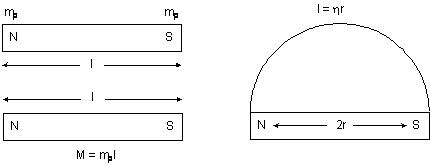(b) The magnetic moment of an electron due to its orbital motion is 1μB whereas that due to its spin motion it is μB /2.

i.e. Morbital =and    Mspin = s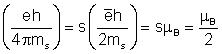Here μB = Bohr magneton

(i) The value of Bohr magneton  μB = eh / 4πm

(ii) μB = 0.93 × 10–23 Amp-m2

(c) Other formula for magnetic moment includes:

(i) M = ni r2

(ii) M = eVr/2 = er2ω/2 = er22πf/2 = er2π/T

(iii)       e/mJ

(iv)       M = nωB

(d) How to calculate resultant magnetic moment:

(i) When two bar magnets are lying mutually perpendicular to each other, then

M = √M12 + M22 = √2mpl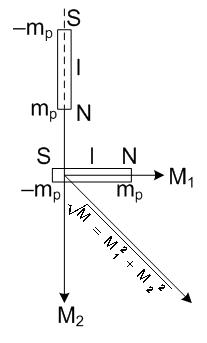(ii) When two coils, each of radius r and carrying current i, are lying concentrically with their planes at right angles to each other, then

M = √M12 + M22 = √2iπr2 if M1 = M2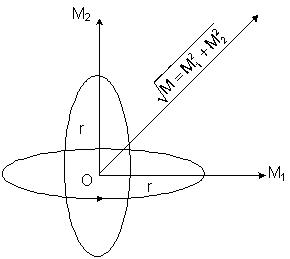Illustration: A square loop OABCO of side l carries a current i. Find the magnetic moment of the loop.

Solution: Magnetic moment of the loop can be written as,

M = i (BC x CO), where the letters in bold denote the vectors

BC = -lkWatch this Video for more reference

Illustration: Find the magnitude of magnetic moment of the current carrying loop ABCDEFA. Each side of the loop is 10 cm long and current in the loop is i = 2.0 A.Solution: By assuming two equal and opposite currents in BE, two current carrying loop (ABEFA and BCDEB) are formed. Their magnetic moments are equal in magnitude but perpendicular to each other. Hence

Mnet = √M2 + M2 = √2 M

Where M = ia = (2.0)(0.1)(0.1) = 0.02 A-m2

Mnet = (√2) (0.02) A – m2 = 0.028 A-m2Illustration: Two identical bar magnets each of length L and pole–strength m are placed at right angles to each other with the north pole of one touching the south pole of the other. Evaluate the magnetic moment of the system.

Solution: As magnetic moment is a vector, so we know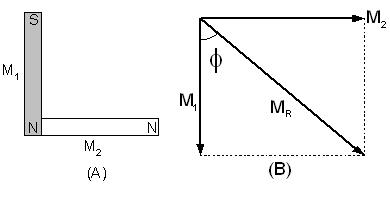MR = (M12 + M22 + 2M1M2 cos θ)1/2  with tanΦ M2 sinθ / M1 + M2 cosθ

and as here M1= M2 = mL and θ = 90°, so

MR = (M2 + M2 + 2MM cos 90)1/2 = (√2) mL

And, tanΦ Msin90 / M + Mcos 90 = 1,   i.e., Φ = tan–1(1) = 45°
The JEE asks tricky questions on magnetic moment and resultant magnetic moment. It is crucial for the JEE aspirants to master various formulae like the resultant magnetic moment formula and the resultant of two magnetic dipole as questions on these topics are often asked.

Related Resources

Refer the Complete syllabus of JEE Physics
Get an idea of the types of questions asked in the past years through our Past Papers and Solutions

Look here for the Best books of Physics
```### Course Features

• 101 Video Lectures
• Revision Notes
• Previous Year Papers
• Mind Map
• Study Planner
• NCERT Solutions
• Discussion Forum
• Test paper with Video Solution